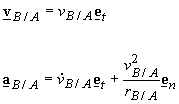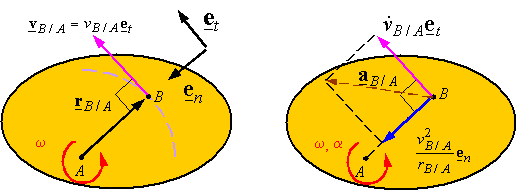Relative motion of points on a rigid body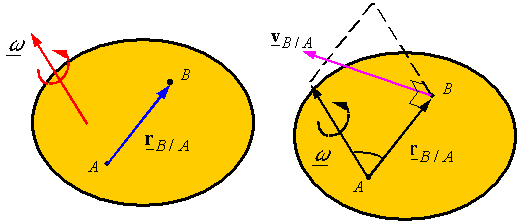Relative velocity of points on a rigid body: Consider points A and B on a rigid body. The relative position of B with respect to A is given by rB/A.

The relative velocity of B with respect to A is given by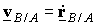. Since the distance between points A and B does not change in a rigid body, |rB/A| is constant and one can calculate the derivative of rB/A using the lemma to get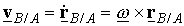where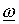is the angular velocity of the rigid body. Since vB/A is the cross product ofand rB/A, the relative velocity of B with respect to A is perpendicular to bothand rB/A.

Relative acceleration of points on a rigid body: The relative acceleration of point B with respect to A is given by the derivative of vB/A. Using the above expression for relative velocity, one gets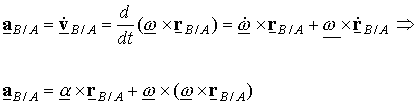2-D motion: In 2-D, the angular velocity and angular acceleration can be written as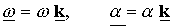The following are graphical representations of the relative velocity and relative acceleration of points on a rigid body.The left figure shows that the motion of  point B relative to A describes a circle since the distance between the points does not change. As a result, the velocity of B relative to A is tangent to this circle. The right figure shows the components of acceleration. Since the relative motion is on a circle, the acceleration of B relative to A has a component tangent to the circle (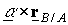= tangential component) and a component towards the center of rotation (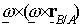= centripetal component).

Since the relative motion of two points on a rigid body always is described by motion on a circular path, one can also use polar coordinates with a constant radial coordinate r (i.e.,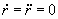),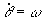, and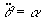.  This results in expressions for relative velocity and acceleration given as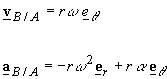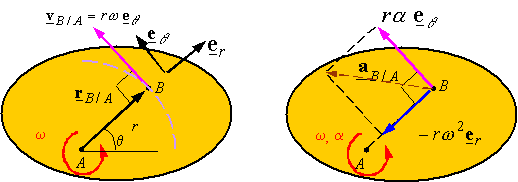Another method of describing relative motion is by using normal and tangential coordinates. This results in